# 2020 HSC Maths Advanced Exam Paper Solutions

In this post, we give you the solutions to the 2020 Maths Advanced paper.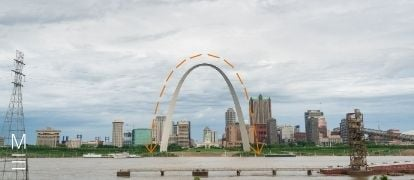The 2020 HSC Mathematics Advanced (2 Unit) exam paper solutions are here!

## 2020 HSC Maths Advanced Exam Paper Solutions

Have you seen the 2020 HSC Mathematics Advanced (2 Unit) exam paper yet?

In this post, we will work our way through the 2020 HSC Maths Advanced (2 Unit) paper and give you the solutions, written by our Head of Mathematics Oak Ukrit and his team.

## Want to see how the Matrix Academic Head of Maths would solve the common questions?

In this video, Head of Maths Oak Ukrit solves the common questions from the 2020 HSC Maths Advanced and Maths Standard 2 papers.

Read on to see how to answer all of the 2020 questions.

## Section 1. Multiple Choice

 Question Number Answer Solution 1. D We can only take the square root of non-negative numbers, so we need $$2x – 3 \geq 0$$. This means that $$2x \geq 3, so \ x \geq \frac{3}{2}$$. 2. B The graph is translated right as we have $$(x \ – \ 2)$$ and the graph is translated up as we have $$+5$$. 3. A \begin{align*} z_{French} \ &= \ \frac{82-70}{8} \ =  \ 1.5 \\ z_{Commerce} \ &= \ \frac{80-65}{5} \ = \ 3 \\ z_{Music} \ &= \ \frac{74-50}{12} \ = \ 2 \end{align*}Comparing z-scores, his weakest subject is French and his strongest subject is Commerce. 4. B $$\int e + e^{3x} \, dx = ex + \frac{1}{3} e^{3x} + c$$, where $$c$$ is a constant. 5. C Here, $$a < 0$$ so the parabola $$y = -x^2 + bx + 1$$ should be concave down. Furthermore, the axis of symmetry is\begin{align*} x &= – \frac{b}{2a} \\ &= -\frac{b}{2 (-1)} \\ &= \frac{b}{2} \\ &> 0 \end{align*}since it is given that $$b > 0$$ . 6. B Since $$-1 \leq \cos 3x \leq 1$$ , and multiplying everything by $$2$$ gives $$-2 \leq 2 \cos 3x \leq 2$$.Adding $$5$$ to everything gives $$5 – 2 \leq 5 + 2 \cos 3x \leq 5 + 2$$ , so $$3 \leq 5 + 2 \cos 3x \leq 7$$ .Therefore, the range of $$5 + 2 \cos 3x$$ is $$[3,7]$$. 7. A \begin{align*} \int_0^{12} f\left(x\right) \, dx &= \text{area under $f\left(x\right)$ from $0$ to $12$} \\ &= 3 \times 4 + 3 \times 4 + \frac{1}{2} \times \pi \times \left(\frac{8 – 4}{2}\right)^2 + \frac{3 \times \left(10 – 8\right)}{2} – \frac{3 \times \left(12 – 10\right)}{2} \\ &= 12 + 12 + 2 \pi + 3 – 3 \\ &= 24 + 2 \pi \,. \end{align*} 8. A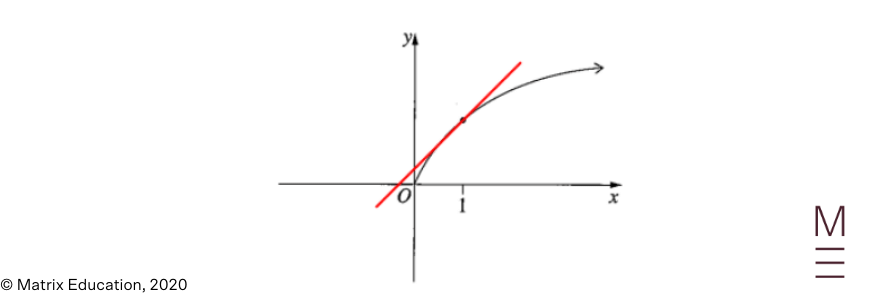The answer is A. We have\begin{align*} f(1) > 0 \end{align*}since it is above the $$x$$ -axis.The graph is concave downwards so\begin{align*} f”(1) < 0 \,. \end{align*}By considering the tangent to $$y = f(x)$$ that passes through the points $$(0, c)$$ and $$(1, f(1))$$, we have\begin{align*} f'(1) &= \text{slope of tangent to $f(x)$ at $x = 1$} \\ &= \frac{f(1) – c}{1 – 0} \\ &= f(1) – c \\ &< f(1) \quad \text{(since we see that} \ c > 0 \ \text{from the graph)} \,. \end{align*}Also, the slope of the tangent to $$f(x)$$ at $$x = 1$$ is positive, so\begin{align*} f'(1) > 0 \,. \end{align*}In conclusion,\begin{align*} f”(1) < 0 < f'(1) < f(1) \,. \end{align*} 9. C We can make the following markings on the normal curves provided: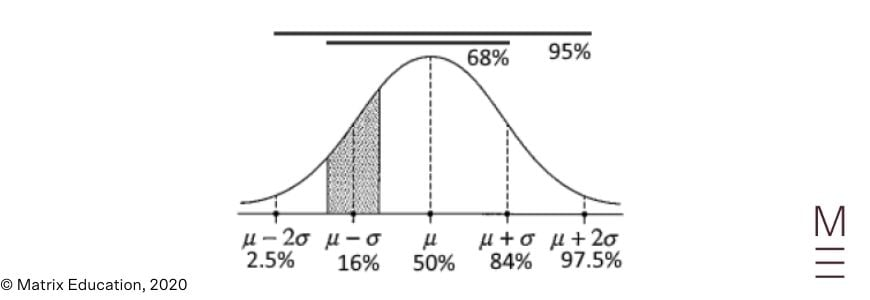Only C then has the lower bound of $$10\%$$ and the upper bound of $$25\%$$ in the correct regions. 10. C Between $$1\le x \le 5, g(x)$$ has a range of $$0 \le g(x) \le 2$$.Between $$0 \le x \le 2$$, $$f(x)$$ has a stationary point at $$1$$.Since  $$g(x)$$ passes through $$1$$ twice, there are two stationary points for $$h(x) = f(g(x))$$ .

## Need extra help answering these questions?## Section 2. Long response

### Question 11a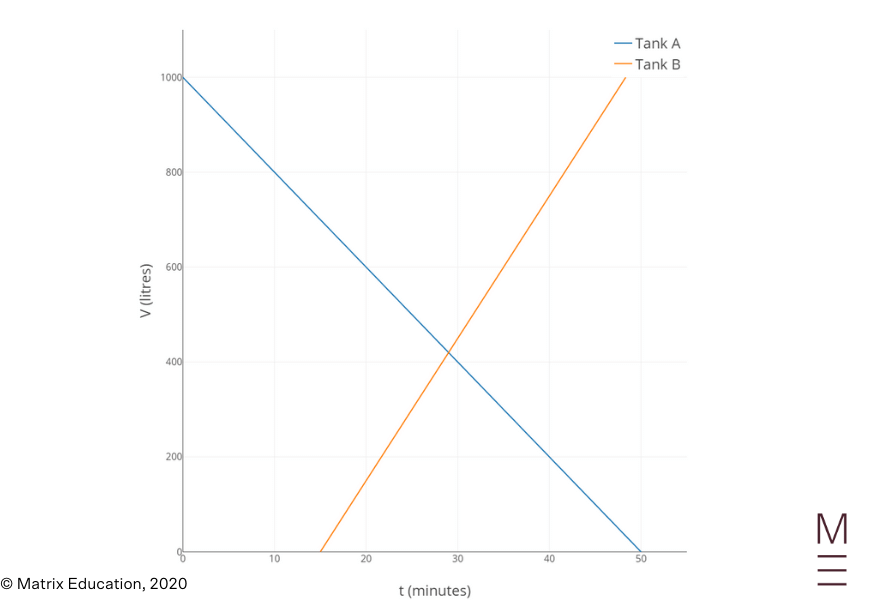### Question 11b

The volume of water in Tank B is $$V = 30t + b$$ . Substituting $$V = 0$$ and $$t = 15$$ into the equation gives

 \begin{align*} 0 &= 30\left(15\right) + b \\ 0 &= 450 + b \\ b &= -450 \ \end{align*}

This means that the volume of water in Tank B is

 $$V = 30t – 450$$

It is given that the volume of water in Tank A is

 $$V = 1000 – 20t$$

So the two tanks have the same volume of water when

 \begin{align*} 30t – 450 &= 1000 – 20t \\ 1450 &= 50t \\ t &= \frac{1450}{50} \\ t &= 29 \end{align*}

Therefore, at $$t = 29$$ minutes, they contain the same volume of water.

### Question 11c

When the total volume of water in the two tanks is $$1000$$ litres, we have

 \begin{align*} (1000 – 20t) + (30t – 450) &= 1000 \\ 550 + 10t &= 1000 \\ t &= \frac{1000 – 550}{10} \\ t &= 45 \end{align*}

Therefore, at $$t = 45$$ minutes, the total volume of water in the two tanks is $$1000$$ litres.

### Question 12

The common difference is

 \begin{align*} d &= T_2 – T_1 \\ &= 10 – 4 \\ &= 6 \end{align*}

The first term is

 $$a = 4$$

Using

 $$T_n = a + (n – 1) d$$

we have

 \begin{equation*} 1354 = 4 + (n – 1) (6) \,, \end{equation*}

so

 \begin{align*} n &= \frac{1354 – 4}{6} + 1 \\ &= 226 \end{align*}

Therefore, the sum of the arithmetic series is

 \begin{align*} S_{226} &= \frac{226}{2} (4 + 1354) \\ &= 153454 \end{align*}

### Question 13

We have

 \begin{align*} \int_0^{\pi/4} \sec^2 x \, dx &= \left[\tan x\right]_0^{\pi/4} \\ &= \tan \left(\frac{\pi}{4}\right) – \tan \left(0\right) \\ &= 1 – 0 \\ &= 1 \ \end{align*}

### Question 14a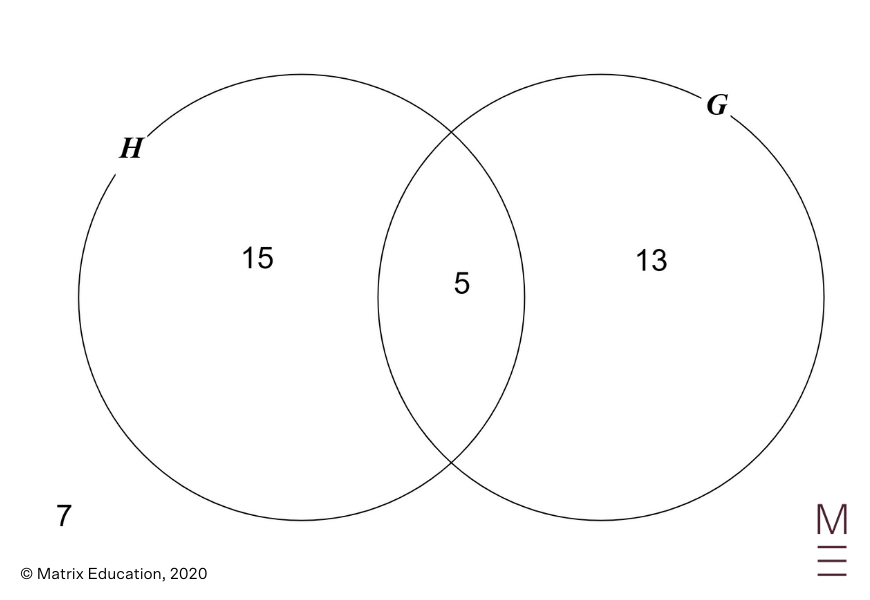We have

 \begin{align*} n \left(H \cup G\right) &= n \left(H\right) + n \left(G\right) – n\left(H \cap G\right) \\ 33 &= 20 + 18 – n \left(H \cap G\right) \\ n\left(H \cap G\right) &= 5 \,. \end{align*}

Therefore,

 \begin{align*} P\left(H \cap G\right) &= \frac{5}{40} \\ &= \frac{1}{8} \,. \end{align*}

### Question 14b

We have

 \begin{align*} P \left(\overline{H} \,|\, G\right) &= \frac{P\left(\overline{H} \cap G\right)}{P\left(G\right)} \\ &= \frac{13}{18} \,. \end{align*}

### Question 14c

The probability required is

 \begin{align*} P\left(H\right) \times P\left(\overline{H}\right) &= \frac{20}{40} \times \frac{20}{39} \\ &= \frac{10}{39} \,. \end{align*}

### Question 15a

We have

 \begin{align*} \angle APB &= \angle NPB – \angle NPA \\ &= 100º – 35º \\ &= 65º \end{align*}

as required.

### Question 15b

Using the cosine rule, we have

 \begin{align*} AB^2 &= AP^2 + PB^2 – 2 \times AP \times PB \times \cos 65º \\ &= 7^2 + 9^2 – 2 \times 7 \times 9 \times \cos 65º  \\ &= 76.75 \end{align*}

so

 \begin{align*} AB &= \sqrt{76.75} \\ &= 8.76 \ \text{km} \quad \text{(correct to 2 d.p.)} \end{align*}

### Question 15c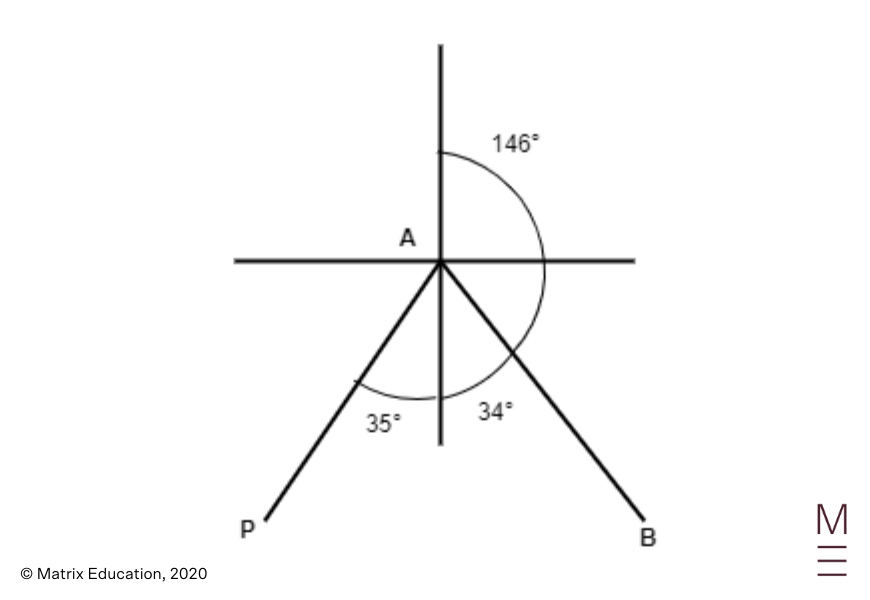Using the cosine rule, we have

 \begin{equation*} PB^2 = AP^2 + AB^2 – 2 \times AP \times AB \times \cos (\angle PAB) \end{equation*}

Rearranging this gives

 \begin{align*} \cos (\angle PAB) &= \frac{AP^2 + AB^2 – PB^2}{2 \times AP \times AB} \\ &= \frac{7^2 + 76.75 – 9^2}{2 \times 7 \times 8.76} \\ &= 0.365 \end{align*}

so

 \begin{align*} \angle PAB &= \cos^{-1} \left(0.365\right) \\ &= 68º 36′ \\ &\approx 69º \end{align*}

Therefore,

 \begin{align*} \text{bearing} &= 180º – (69º – 35º) \\ &= 146º \quad \text{(correct to the nearest degree)} \,. \end{align*}

### Question 16

Calculating the derivatives gives

 \begin{align*} \frac{dy}{dx} &= \frac{d}{dx} (-x^3 + 3x^2 – 1) \\ &= -3x^2 + 6x \\ &= -3x (x – 2) \end{align*}

and

 \begin{align*} \frac{d^2 y}{dx^2} & = \frac{d}{dx} (-3x^2 + 6x) \\ & = -6x + 6 \\ & = -6(x – 1) \end{align*}

For the stationary points, setting $$\frac{dy}{dx} = 0$$ gives

 $$-3x (x – 2) = 0$$

so

 \begin{align*} x = 0 \quad \text{or} \quad x = 2. \end{align*}

When $$x = 0$$,

 \begin{align*} y &= -0^3 + 3 (0)^2 – 1 \\ &= -1 \end{align*}

When $$x = 2$$

 \begin{align*} y &= -2^3 + 3(2)^2 – 1 \\ &= 3 \end{align*}

Therefore, the stationary points are $$(0,-1)$$ and $$(2, 3)$$.

 $$x$$ $$x < 0$$ $$x = 0$$ $$0 < x < 2$$ $$x = 2$$ $$x > 2$$ $$\frac{dy}{dx}$$ $$–$$ $$0$$ $$+$$ $$0$$ $$–$$

For the points of inflection, setting $$\frac{d^2 y}{dx^2} = 0$$ gives

 \begin{align*} -6(x – 1) = 0 \end{align*}

So,

 \begin{align*} x = 1 \end{align*}

When $$x = 1$$,

 \begin{align*} y &= -1^3 + 3(1)^2 – 1 \\ &= 1 \end{align*}

Therefore, the point of inflection is $$(1,1)$$.

 $$x$$ $$x < 1$$ $$x = 0$$ $$x > 1$$ $$\frac{d^{2}y}{dx^{2}}$$ $$+$$ $$0$$ $$–$$

The $$y$$ -intercept is

 \begin{align*} y &= -0^3 + 3(0)^2 – 1 \\ &= -1 \end{align*}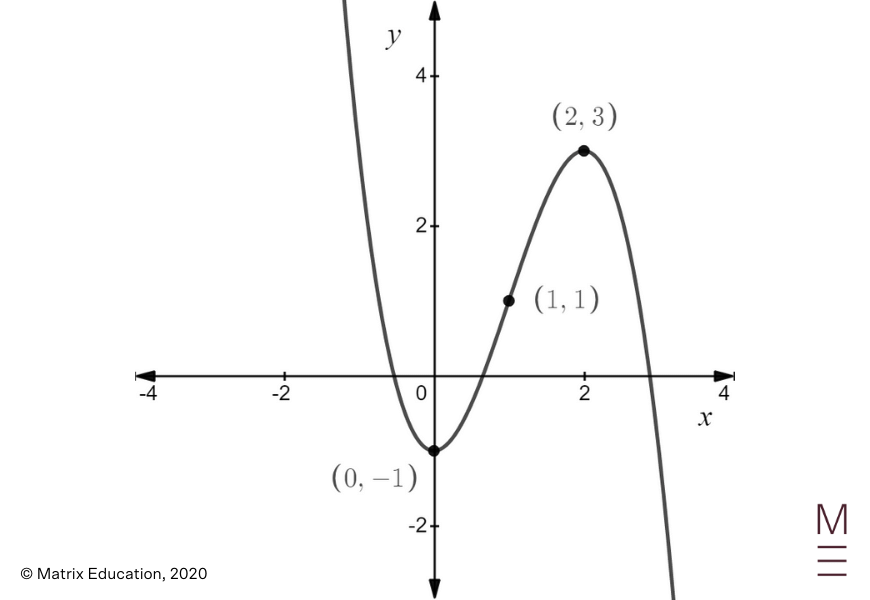### Question 17

We use integration by substitution. Let $$u = 4 + x^2$$. Then $$\frac{du}{dx} = 2x$$, so $$du = 2x \ dx$$.

Therefore,

 \begin{align*} \int \frac{x}{4 + x^2} \, dx &= \int \frac{1}{2} \left(\frac{2x}{4 + x^2}\right) \, dx \\ &= \int \frac{1}{2} \left(\frac{du}{u}\right) \\ &= \frac{1}{2} \ln \left|u\right| + C \\ &= \frac{1}{2} \ln \left|4 + x^2\right| + C \\ &= \frac{1}{2} \ln \left(4 + x^2\right) + C \,, \quad \text{since $4 + x^2 > 0$} \,, \end{align*}

Where $$C$$ is a constant.

### Question 18a

Using the product rule gives

 \begin{align*} \frac{d}{dx} \left(e^{2x} \left(2x + 1\right)\right) &= e^{2x} \frac{d}{dx} \left(2x + 1\right) + \left(2x + 1\right) \frac{d}{dx} \left(e^{2x}\right) \\ &= e^{2x} \left(2\right) + \left(2x + 1\right) \left(2e^{2x}\right) \\ &= 2e^{2x} + 4xe^{2x} + 2e^{2x} \\ &= 4e^{2x} + 4xe^{2x} \\ &= 4e^{2x} \left(1 + x\right) \,. \end{align*}

### Question 18b

We have

 \begin{align*} \int \left(x + 1\right) e^{2x} \, dx &= \frac{1}{4} \int 4 \left(x + 1\right) e^{2x} \, dx \\ &= \frac{1}{4} \left[e^{2x} \left(2x + 1\right)\right] + C \,, \end{align*}

where $$C$$ is a constant, using the result from part (a).

## Need extra help answering these questions?### Question 19

Starting from the left hand side, we have

 \begin{align*} \sec \theta – \cos \theta &= \frac{1}{\cos \theta} – \cos \theta \\ &= \frac{1 – \cos^2 \theta}{\cos \theta} \\ &= \frac{\sin^2 \theta}{\cos \theta} \\ &= \sin \theta \cdot \frac{\sin \theta}{\cos \theta} \\ &= \sin \theta \tan \theta \end{align*}

as required.

### Question 20

Using the trapezoidal rule, we find that

 \begin{align*} \text{approximate distance} &= \frac{\frac{1}{60}}{2} \left[60 + 2 \left(55 + 65 + 68 + 70\right) + 67\right] \\ &= \frac{643}{120} \\ &= 5.358 \end{align*}

### Question 21a

The temperate of the tea 4 minutes after it has been poured is found by substituting $$t = 4$$ into $$T$$ , which gives

 \begin{align*} T &= 25 + 70 \left(1.5\right)^{-0.4 \left(4\right)} \\ &= 25 + 70 \left(1.5\right)^{-0.4 \left(4\right)} \\ &= 25 + 70\left(1.5\right)^{-1.6} \\ &\approx 61.5891 \text{degrees Celsius.} \end{align*}

### Question 21b

The rate of change of the temperature of the tea is

 \begin{align*} \frac{dT}{dt} &= \frac{d}{dt} \left(25 + 70\left(1.5\right)^{-0.4t}\right) \\ &= 70\left(1.5\right)^{-0.4t} \left(\log_e 1.5\right) \frac{d}{dt} \left(-0.4t\right) \\ &= 70\left(1.5\right)^{-0.4t} \left(\log_e 1.5\right) \left(-0.4\right) \\ &= \left(-28\log_e 1.5\right) \left(1.5\right)^{-0.4t} \,. \end{align*}

When $$t = 4$$ , this becomes

 \begin{align*} \frac{dT}{dt} &= \left(-28\log_e 1.5\right) \left(1.5\right)^{-0.4 \left(4\right)} \\ &\approx -5.93425 \,, \end{align*}

which is negative, so the tea is cooling at the rate of $$5.93425$$ degrees Celsius per minute.

### Question 21c

Substituting $$T = 55$$ into $$T = 25 + 70 (1.5)^{-0.4t}$$ gives

 \begin{equation*} 55 = 25 + 70 \left(1.5\right)^{-0.4t} \,, \end{equation*}

so

 \begin{equation*} 30 = 70 \left(1.5\right)^{-0.4t} \,, \end{equation*}

and

 \begin{equation*} \frac{3}{7} = \left(1.5\right)^{-0.4t} \,. \end{equation*}

Taking logarithms on both sides of the equation gives

 \begin{equation*} \log_e \left(\frac{3}{7}\right) = \log_e \left(1.5\right)^{-0.4t} \,, \end{equation*}

so

 \begin{equation*} \log_e \left(\frac{3}{7}\right) = -0.4t \log_e \left(1.5\right) \,, \end{equation*}

and

 \begin{align*} t &= -\frac{1}{0.4 \log_e \left(1.5\right)} \log_e \left(\frac{3}{7}\right) \\ &\approx 5.22423 \end{align*}

### Question 22

Using the given perimeter we have

 \begin{align*} AB &= \frac{\text{perimeter}}{\text{number of sides}} \\ &= \frac{80}{10} \\ &= 8 \, \textrm{cm} \end{align*}

We also have

 \begin{align*} \angle AOB &= 360° \div 10 \\ &= 36° \end{align*}

Using the cosine rule gives

 $$AB^2 = AO^2 + BO^2 – 2 \times AO \times BO \times \cos \angle AOB$$

Since $$AO = BO$$ and $$AB = 8$$, we have

 \begin{align*} 8^2 &= AO^2 + AO^2 – 2 \times AO \times AO \times \cos 36° \\ 64 &= 2 (AO)^2 – 2 AO^2 \cos 36° \\ 64 &= AO^2  (2 – 2 \cos 36°) \\ AO^2 &= \frac{64}{2 – 2 \cos 36°} \end{align*}

Therefore,

 \begin{align*} \text{area of the decagon} &= 10 \times \text{area of} \triangle \text{AOB} \\ &= 10 \times \left[\frac{1}{2} \times AO^2 \sin 36º \right] \\ &= 10 \times \frac{1}{2} \times \frac{64}{2 – 2 \cos 36º} \times \sin 36º \\ &= 492.429 \\ &\approx 492.4 \, \textrm{cm}^2 \quad \text{(correct to 1 d.p.)} \end{align*}

### Question 23a

Since $$f(x)$$ is a probability density function, we know that

 \begin{align*} \int_0^k \sin x \, dx &= 1 \\ \left[-\cos x\right]_0^k &= 1 \\ – \cos k – \left(- \cos 0\right) &= 1 \\ – \cos k + 1 &= 1 \\ \cos k &= 0 \, \end{align*}

Solving this for $$k$$ gives

 $$k = \frac{\pi}{2}$$

### Question 23b

Since $$1 < \frac{\pi}{2}$$, we have

 \begin{align*} P\left(X \leq 1\right) &= \int_0^1 f\left(x\right) \, dx \\ &= \int_0^1 \sin x \, dx \\ &= \left[-\cos x\right]_0^1 \\ &= -\cos 1 – \left(- \cos 0\right) \\ &= – \cos 1 + 1 \\ &= 0.4597 \,. \end{align*}

### Question 24

Starting with the circle given, we complete the square and obtain

 \begin{align*} x^2 – 6x + y^2 + 4y – 3 &= 0 \\ x^2 – 6x + y^2 + 4y &= 3 \\ \left(x^2 – 6x + 9\right) + \left(y^2 + 4y + 4\right) &= 3 + 9 + 4 \\ \left(x – 3\right)^2 + \left(y + 2\right)^2 &= 16 \ \end{align*}

This means that the original circle is centred at $$(3, -2)$$ and its radius is $$4$$ units.

When it is reflected in the $$x$$ -axis, we multiply the $$y$$ -coordinate by $$-1$$ so the centre of the reflected circle is $$(3,2)$$ , and the radius is still $$4$$ units.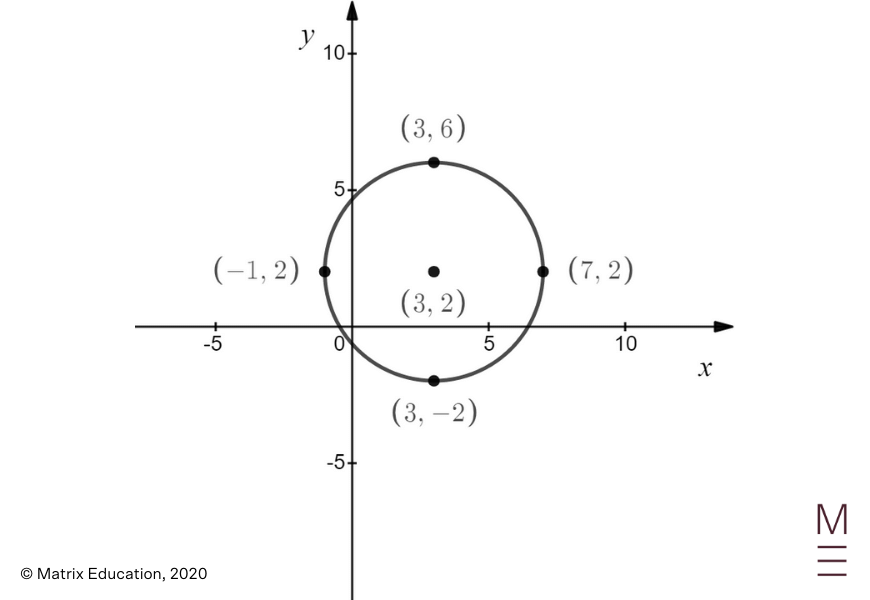### Question 25a

The radius of the quarter circle is $$x$$ metres. We have

 \begin{align*} \text{area of garden bed} &= \text{area of rectangle} + \text{area of quarter circle} \\ &= xy + \frac{1}{4} \left(\pi x^2\right) \,. \end{align*}

Since this has to be equal to $$36$$, we have

 \begin{align*} xy + \frac{1}{4} \left(\pi x^2\right) &= 36 \\ xy &= 36 – \tfrac{1}{4} \left(\pi x^2\right) \\ y &= \frac{36}{x} – \frac{\pi x}{4} \,. \end{align*}

Therefore, the perimeter is given by

 \begin{align*} P &= x + y + \left(x + y\right) + \frac{1}{4} \left(2 \pi x\right) \\ &= 2 \left(x + y\right) + \frac{\pi x}{2} \\ &= 2 \left[x + \left(\frac{36}{x} – \frac{\pi x}{4}\right)\right] + \frac{\pi x}{2} \\ &= 2x + \frac{72}{x} – \frac{\pi x}{2} + \frac{\pi x}{2} \\ &= 2x + \frac{72}{x} \,, \end{align*}

as required.

### Question 25b

First, we differentiate $$P = 2x + \frac{72}{x}$$ and obtain

 \begin{align*} \frac{dP}{dx} &= \frac{d}{dx} \left(2x + \frac{72}{x}\right) \\ &= 2 – \frac{72}{x^2} \,. \end{align*}

Setting $$\frac{dP}{dx} = 0$$ gives $$2 – \frac{72}{x^2} = 0$$. This means that

 $$\frac{72}{x^2} = 2$$

so

 $$x^2 = 36$$

and

 $$x = 6$$

since $$x$$ is the dimension of a rectangle and it must be non-negative. When $$x = 6$$, the perimeter is given by

 \begin{align*} P &= 2 \left(6\right) + \frac{72}{6} \\ &= 12 + 12 \\ &= 24 \ \text{metres} \end{align*}
 $$x$$ $$0 < x < 6$$ $$x = 6$$ $$x > 6$$ $$\frac{dP}{dx}$$ $$–$$ $$0$$ $$+$$

Therefore, $$P = 24$$ is the smallest possible perimeter.

### Question 26a

We use the recurrence relation starting with

 $$A_0 = 60000$$

The amount of money in the account after the first withdrawal is

 \begin{align*} A_1 &= A_0 \left(1.005\right) – 800 \\ &= 60000 \left(1.005\right) – 800 \,, \end{align*}

The amount of money in the account after the second withdrawal is

 \begin{align*} A_2 &= A_1 \left(1.005\right) – 800 \\ &= \left[60000 \left(1.005\right) – 800\right] \left(1.005\right) – 800 \\ &= 60000 \left(1.005\right)^2 – 800 \left(1 + 1.005\right) \,, \end{align*}

And the amount of money in the account after the third withdrawal is

 \begin{align*} A_3 &= A_2 \left(1.005\right) – 800 \\ &= \left[60000 \left(1.005\right)^2 – 800 \left(1 + 1.005\right)\right] \left(1.005\right) – 800 \\ &= 60000 \left(1.005\right)^3 – 800 \left(1 + 1.005 + 1.005^2\right) \\ &\approx 58492.49 \quad \text{(correct to 2 d.p.)} \,. \end{align*}

### Question 26b

The interest in the first month is

 \begin{align*} I_1 &= A_0 \times 0.5\% \\ &= 60000 \times 0.005 \\ &= \300 \end{align*} The interest in the second month is  \begin{align*} I_2 &= A_1 \times 0.5\% \\ &= \left(60000 \times 1.005 – 800\right) \times 0.005 \\ &= \ 297.50 \end{align*}

The interest in the third month is

 \begin{align*} I_3 &= A_2 \times 0.5\% \\ &= \left(60000 \times 1.005^2 – 800 \times 1.005 – 800\right) \times 0.005 \\ &= \294.99 \end{align*} Therefore, the total amount of interest earned in the first three months is  \begin{align*} I_1 + I_2 + I_3 &= \ 892.49 \end{align*}

### Question 26c

The amount of money in the account immediately after the 94th withdrawal is

### Question 29a

Since

 \begin{align*} \frac{dy}{dx} &= \frac{d}{dx} \left(c \ln x\right) \\ &= \frac{c}{x} \,, \end{align*}

the slope of the tangent to $$y = c \ln x$$ at $$x = p$$ is

 $$\frac{dy}{dx}\bigg|_{x = p} = \frac{c}{p}$$

When $$x = p$$, the corresponding $$y$$-coordinate is

 \begin{equation*} y = c \ln p \\ \end{equation*}

This means that the equation of the tangent to $$y = c \ln x$$ at  $$x = p$$ is given by

 \begin{equation*} \frac{y – c \ln p}{x – p} = \frac{c}{p} \end{equation*}

Multiplying both sides by $$x – p$$ gives $$y – c \ln p = \frac{c}{p} \left(x – p\right)$$, so

 \begin{align*} y &= \frac{c}{p} \left(x – p\right) + c \ln p \\ &= \frac{c}{p} x – c + c \ln p \,, \end{align*}

as required.

### Question 29b

Since the tangent has a gradient of $$1$$, we have

 \begin{equation} \label{eq:Q29bEq1} \frac{c}{p} = 1 \end{equation}

which means that

 \begin{equation} \label{eq:Q29bEq2} c = p \end{equation}

Furthermore, since the tangent passes through the origin, substituting $$x = 0$$ and $$y = 0$$ into the equation of the tangent gives

 \begin{equation*} 0 = \frac{c}{p} \left(0\right) – c + c \ln p \end{equation*}

which means that $$0 = -c + c \ln p$$, or equivalently,

 \begin{equation} \label{eq:Q29bEq3} c \left(-1 + \ln p\right) = 0 \end{equation}

From Eq. $$\frac{c}{p} = 1$$, we know that $$c \neq 0$$, so Eq. $$c \left(-1 + \ln p\right) = 0$$ implies that

 \begin{equation*} -1 + \ln p = 0 \,, \end{equation*}

which means that

 \begin{equation*} \ln p = 1 \,, \end{equation*}

and

 \begin{equation} \label{eq:Q29bEq4} p = e \ \end{equation}

Substituting $$p = e$$ into $$c = p$$ gives

 \begin{equation*} c = e \,. \end{equation*}

### Question 30a

Since $$A$$ is the point of intersection of

 \begin{equation*} y = 4x – x^2 \end{equation*}

and

 \begin{equation*} y = ax^2 \end{equation*}

its $$x$$ -coordinate is found by equating both equations and solving for $$x$$. This gives

 \begin{equation*} 4x – x^2 = ax^2 \end{equation*}

so

 \begin{equation*} \left(1 + a\right) x^2 – 4x = 0 \end{equation*}

or equivalently,

 \begin{equation*} x \left [ \left(1 + a \right )x – 4 \right] = 0 \end{equation*}

Either $$x = 0$$ or $$\left(1 + a\right) x – 4 = 0$$, but from the diagram we see that the $$x$$ coordinate of  $$A$$ is not $$0$$, which means that we must have

 \begin{equation*} \left(1 + a\right) x – 4 = 0 \end{equation*}

Rearranging this gives

 \begin{equation*} \left(1 + a\right) x = 4 \end{equation*}

so

 \begin{equation*} x = \frac{4}{1 + a} \end{equation*}

as required.

### Question 30b

The shaded area is given by

 \begin{align*} \int_0^{4/\left(1+a\right)} \left[\left(4x – x^2\right) – ax^2\right] \, dx &= \int_0^{4/\left(1+a\right)} \left[\left(4x – x^2\right) – ax^2\right] \, dx \\ &= \int_0^{4/\left(1+a\right)} \left(4x – x^2 – ax^2\right) \, dx \\ &= \left[2x^2 – \frac{x^3}{3} – \frac{ax^3}{3}\right]_0^{4/\left(1+a\right)} \\ &= 2\left(\frac{4}{1 + a}\right)^2 – \frac{1}{3} \left(\frac{4}{1 + a}\right)^3 – \frac{a}{3} \left(\frac{4}{1 + a}\right)^3 \\ &= \frac{32}{\left(1 + a\right)^2} – \frac{64}{3 \left(1 + a\right)^3} – \frac{64a}{3\left(1 + a\right)^3} \\ &= \frac{96 \left(1 + a\right) – 64 -64a}{3\left(1 + a\right)^3} \\ &= \frac{96 + 96a – 64 -64a}{3\left(1 + a\right)^3} \\ &= \frac{32 \left(1 + a\right)}{3\left(1 + a\right)^3} \\ &= \frac{32}{3\left(1 + a\right)^2} \end{align*}

Therefore, in order for the shaded area to be equal to $$\frac{16}{3}$$, we need

 \begin{equation*} \frac{32}{3\left(1 + a\right)^2} = \frac{16}{3} \end{equation*}

which means

 \begin{equation*} \left(1 + a\right)^2 = 2 \end{equation*}

Therefore,

 \begin{equation*} 1 + a = \pm \sqrt{2} \end{equation*}

so $$a = -1 \pm \sqrt{2}$$. From the diagram, we need $$a > 0$$, so

 \begin{equation*} a = -1 + \sqrt{2} \end{equation*}

### Question 31a

$$a$$ represents the amplitude, so

 \begin{align*} a &= \frac{35000 – 5000}{2} \\ &= 15000 \end{align*}

$$b$$ represents the vertical displacement, so

 \begin{align*} b &= 35000 – 15000 \\ &= 20000 \end{align*}

### Question 31b

Differentiating $$m\left(t\right)$$ gives

 \begin{equation*} m’\left(t\right) = 15000\left(\frac{\pi}{26}\right) \cos \left(\frac{\pi}{26} t\right) \end{equation*}

For $$m\left(t\right)$$ to be increasing, we assess when $$m’\left(t\right) > 0$$. This occurs when

 \begin{equation*} \cos \left(\frac{\pi}{26} t\right) > 0 \end{equation*}

So

 \begin{equation*} \frac{\pi}{26} t < \frac{\pi}{2} \quad \text{or} \quad \frac{\pi}{26} t > \frac{3 \pi}{2} \end{equation*}

This means that

 \begin{equation*} t < 13 \quad \text{or} \quad t > 39 \end{equation*}

Likewise with $$c\left(t\right)$$, and we have

 \begin{equation*} c’\left(t\right) = \frac{80 \pi}{26} \sin \left[\frac{\pi}{26} \left(t – 10\right)\right] \end{equation*}

Now, $$c’\left(t\right) > 0$$ occurs when

 \begin{equation*} \sin \left[\frac{\pi}{26} \left(t – 10\right)\right] > 0 \end{equation*}

which means that

 \begin{equation*} 0 < \frac{\pi}{26} \left(t – 10\right) < \pi \end{equation*}

so

 \begin{equation*} 0 < t < 36 \end{equation*}

Therefore, both populations are increasing when

 \begin{equation*} 0 < t < 13 \end{equation*}

### Question 31c

First, we find when $$c\left(t\right)$$ reaches a maximum. This occurs when $$c’\left(t\right) = 0$$, so

 \begin{equation*} \frac{80 \pi}{26} \sin \left[\frac{\pi}{26} \left(t – 10\right)\right] = 0 \,, \end{equation*}

or equivalently,

 \begin{equation*} \sin \left[\frac{\pi}{26} \left(t – 10\right)\right] = 0 \,. \end{equation*}

Therefore,

 \begin{equation*} \frac{\pi}{26} \left(t – 10\right) = 0 \quad \text{or} \quad \frac{\pi}{26} \left(t – 10\right) = \pi \,, \end{equation*}

and the stationary points are

 \begin{equation*} t = 10 \quad \text{or} \quad t = 36 \,. \end{equation*}

To determine the nature of these stationary points, we examine $$c”\left(t\right)$$. Now,

 \begin{equation*} c”\left(t\right) = \frac{80 \pi^2}{26^2} \cos \left(\frac{\pi}{26} \left(t – 10\right)\right) \,. \end{equation*}

At $$t = 36$$,

 \begin{align*} c”\left(36\right) &= -1.168 \\ &< 0 \end{align*}

so $$c\left(t\right)$$ attains its maximum when $$t = 36$$. At $$t = 36$$ ,

 \begin{align*} m’\left(36\right) &= \frac{15000}{26} \cos \left(\frac{36 \pi}{26}\right) \\ &= -204.58 \\ \end{align*}

## Want to know your ATAR?

Use the calculator to explore the HSC Marks you need to achieve your ATAR Goal.### Get free study tips and resources delivered to your inbox.

Join 75,893 students who already have a head start.

Our website uses cookies to provide you with a better browsing experience. If you continue to use this site, you consent to our use of cookies. Read our cookies statement.